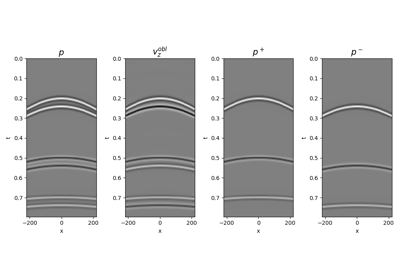# pylops.waveeqprocessing.PressureToVelocity¶

pylops.waveeqprocessing.PressureToVelocity(nt, nr, dt, dr, rho, vel, nffts=(None, None, None), critical=100.0, ntaper=10, topressure=False, backend='numpy', dtype='complex128')[source]

Pressure to Vertical velocity conversion.

Apply conversion from pressure to vertical velocity seismic wavefield (or vertical velocity to pressure). The input model and data required by the operator should be created by flattening the a wavefield of size $$(\lbrack n_{r_y} \times n_{r_x} \times n_t \rbrack$$.

Parameters: nt : int Number of samples along the time axis nr : Number of samples along the receiver axis (or axes) dt : float Sampling along the time axis dr : Sampling(s) along the receiver array rho : float Density $$\rho$$ along the receiver array (must be constant) vel : float Velocity $$c$$ along the receiver array (must be constant) nffts : tuple, optional Number of samples along the wavenumber and frequency axes critical : float, optional Percentage of angles to retain in obliquity factor. For example, if critical=100 only angles below the critical angle $$\sqrt{k_y^2 + k_x^2} < \frac{\omega}{c}$$ will be retained ntaper : float, optional Number of samples of taper applied to obliquity factor around critical angle topressure : bool, optional Perform conversion from particle velocity to pressure (True) or from pressure to particle velocity (False) backend : str, optional Backend used for creation of obliquity factor operator (numpy or cupy) dtype : str, optional Type of elements in input array. Cop : pylops.LinearOperator Pressure to particle velocity (or particle velocity to pressure) conversion operator

UpDownComposition2D
2D Wavefield composition
UpDownComposition3D
3D Wavefield composition
WavefieldDecomposition
Wavefield decomposition

Notes

A pressure wavefield $$p(x, t)$$ can be converted into an equivalent vertical particle velocity wavefield $$v_z(x, t)$$ by applying the following frequency-wavenumber dependant scaling :

$\hat{v}_z(k_x, \omega) = \frac{k_z}{\omega \rho} \hat{p}(k_x, \omega)$

where the vertical wavenumber $$k_z$$ is defined as $$k_z=\sqrt{\frac{\omega^2}{c^2} - k_x^2}$$.

Similarly a vertical particle velocity can be converted into an equivalent pressure wavefield by applying the following frequency-wavenumber dependant scaling :

$\hat{p}(k_x, \omega) = \frac{\omega \rho}{k_z} \hat{v}_z(k_x, \omega)$

For 3-dimensional applications the only difference is represented by the vertical wavenumber $$k_z$$, which is defined as $$k_z=\sqrt{\frac{\omega^2}{c^2} - k_x^2 - k_y^2}$$.

In both cases, this operator is implemented as a concatanation of a 2 or 3-dimensional forward FFT (pylops.signalprocessing.FFT2 or pylops.signalprocessing.FFTN), a weighting matrix implemented via pylops.basicprocessing.Diagonal, and 2 or 3-dimensional inverse FFT.

  (1, 2) Wapenaar, K. “Reciprocity properties of one-way propagators”, Geophysics, vol. 63, pp. 1795-1798. 1998.

## Examples using pylops.waveeqprocessing.PressureToVelocity¶14. Seismic wavefield decomposition